# Worksheets of volume units conversion

Do you want to convert volume units?

 Number of examples: Range of values: Multiplicity:
 15 cl = l 36 cm3 = dl 56 cl = l 81 l = cm3 36 cl = m3 45 dm3 = cl 16 dm3 = m3 35 dl = mm3 101 cm3 = m3 11 m3 = km3

### Conversions of of a volume units in word problems

• Cylinder - h2Cylinder volume is 2.6 liters. Base area is 1.3 dm2. Calculate the height of the cylinder.
• Copper sheetThe copper plate has a length of 1 m, width 94 cm and weighs 9 kg. What is the plate thickness, if 1 m3 weighs 8715 kg?
• Converting units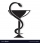Health care professionals frequently convert from one system of measurement to another. Whether you are converting a metric measurement into the household system for a patient or converting within the metric system to enter data in a specific format, the
• Reservoir 3How many cubic feet of water is stored in the reservoir that has a length of 200 feet, a width of 100 feet, an overflow depth of 32 feet, and a current water level of 24 feet?
• Two cuboidsFind the volume of the cuboidal box whose one edge is: a) 1.4m and b) 2.1 dm.
• Permille of alcohol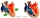I have 2 per mille of alcohol in my blood. How many milliliters is it when I have 5 liters of blood?
• Wood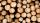Wood density is 0.6 g/cm3. How many kilograms weight 1 m3 of wood?
• Pool 3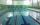How long will fill pool cuboid shape (8m 6m 1.5m) when flows 15 liters/s?
• RainIt rains at night. On 1 m2 of lake will drop 60 liters of water. How many cm will the lake level rise?
• Hemisphere - roof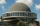The shape of the observatory dome is close to the hemisphere. Its outer diameter is 11 m. How many kilograms of paint and how many liters of thinner is used for its double coat if you know that 1 kg of paint diluted with 1 deciliter of thinner will paint
• Aquarium heightHow high does the water in the aquarium reach, if there are 36 liters of water in it? The length of the aquarium is 60 cm and the width is 4 dm.
• Oak cuboidOak timber is rectangular shaped with dimensions of 2m, 30 cm and 15 cm. It weight is 70 kg. Calculate the weight 1 dm³ of timber.
• Water in aquariumThe aquarium cuboid shape with a length of 25 cm and a width of 30 cm is 9 liters of water. Calculate the areas which are wetted with water.